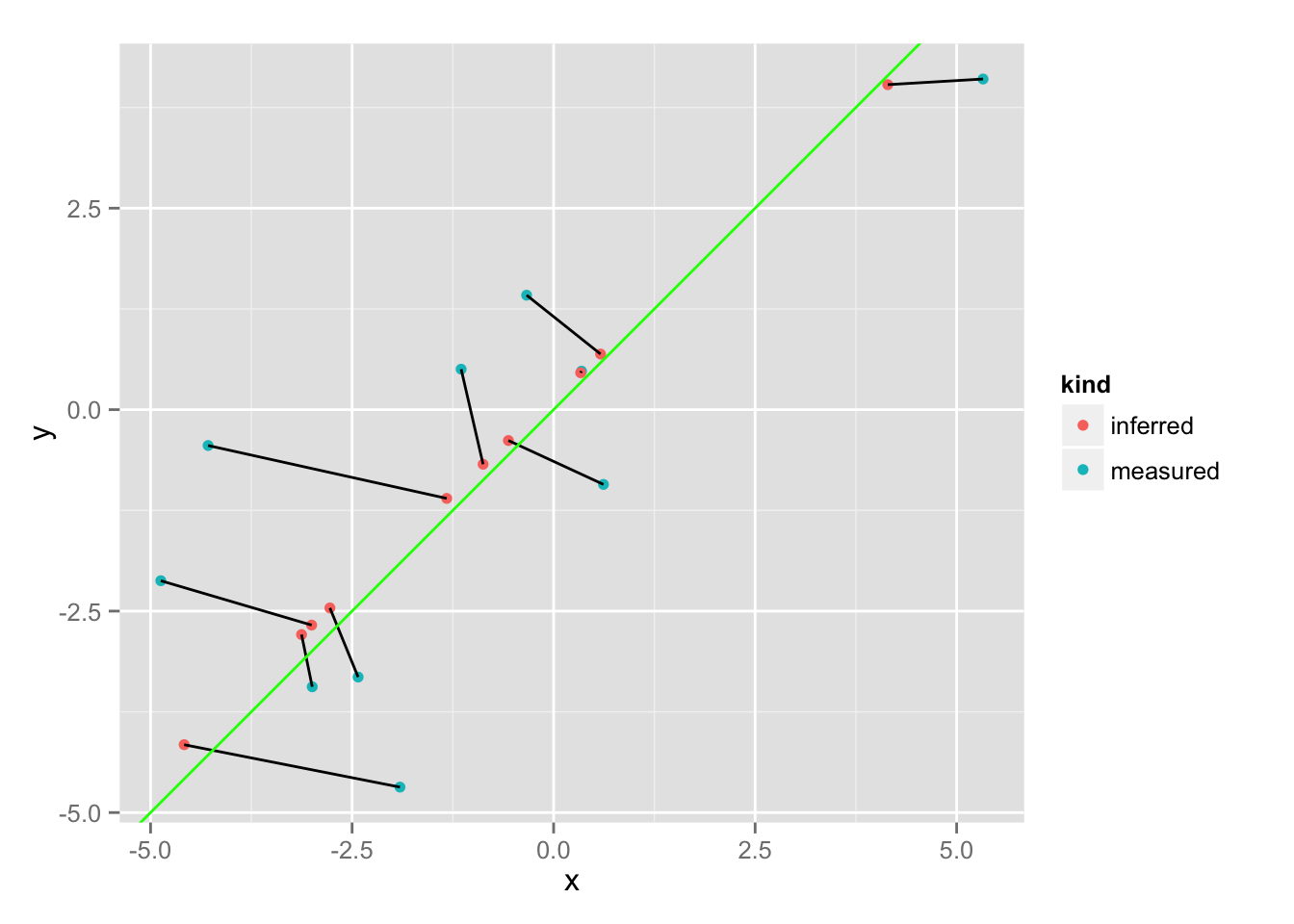## Monday, August 24, 2015

### Fitting with uncertainty using Bayesian inference

This is a mirror of my post on RPubs. The RMarkdown source can be found here.

In this toy example, we assume that we’ve independently measured values \(x\) and \(y\) and want to find a linear relationship between them, accounting for measurement uncertainty. Each \(x\) and \(y\) value is assigned a different uncertainty, and the challenge is to take this information into account. A standard linear model will treat all points equally.

When we treat each measurement as a multivariate normal distribution, we can find a point along the proposed fitted line that is maximizing the probability density function (i.e. that has a maximum likelihood). Thus, given a slope and intercept, we virtually move all measurements to their most likely point along the line, and use the likelihood at this point.

``library(rstan)``
``````## Loading required package: Rcpp
##
## Attaching package: 'inline'
##
## The following object is masked from 'package:Rcpp':
##
##     registerPlugin
##
## rstan (Version 2.7.0-1, packaged: 2015-07-17 18:12:01 UTC, GitRev: 05c3d0058b6a)
## For execution on a local, multicore CPU with excess RAM we recommend calling
## rstan_options(auto_write = TRUE)
## options(mc.cores = parallel::detectCores())``````
``````library(ggplot2)

set.seed(42)

N <- 10``````

We create 10 points:

``````x0 <- seq(-5, 5, 10.1/N)
y0 <- x0

sigma_x <- abs( rnorm( length(x0), 1) )
x <- x0 + unlist(lapply(sigma_x, function(sigma) rnorm(1, 0, sigma)))

sigma_y <- abs( rnorm( length(y0), 1) )
y <- y0 + unlist(lapply(sigma_y, function(sigma) rnorm(1, 0, sigma)))

data <- data.frame( x, sigma_x, y, sigma_y )

data_list <- list( x=x, sigma_x=sigma_x, y=y, sigma_y = sigma_y, N=N)

ggplot( data, aes(x, y) ) + geom_point() + geom_abline(color="red") + geom_smooth(method="lm", se=F) + geom_errorbar(aes(ymin=y-sigma_y, ymax=y+sigma_y)) + geom_errorbarh(aes(xmin=x-sigma_x, xmax=x+sigma_x))``````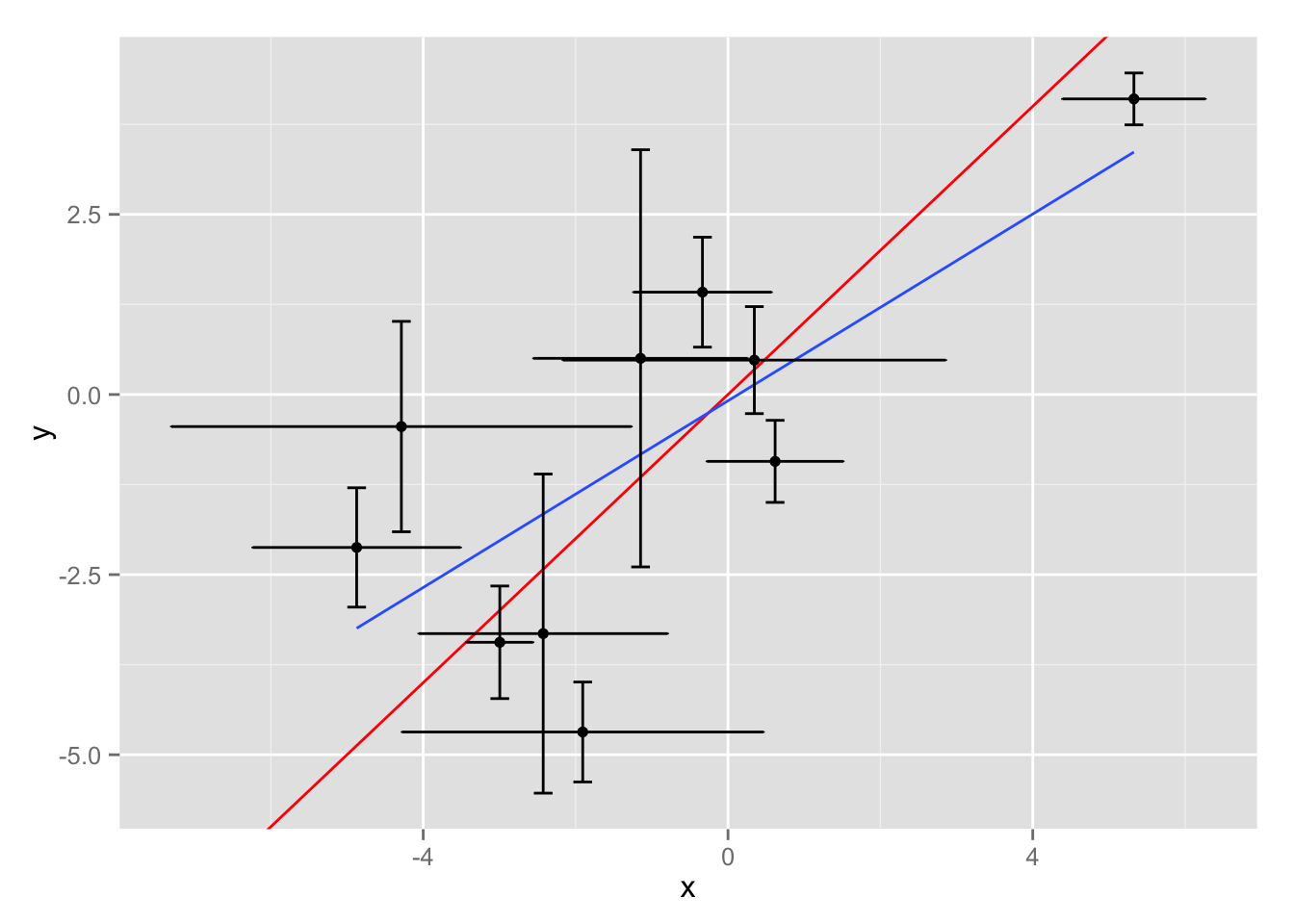(Red: actual dependency, blue: simple linear fit)

The simple linear model in STAN:

``````simple_code <- "
data {
int<lower=0> N;

vector[N] x;
vector[N] y;
}

parameters {
real slope;
real intercept;
real<lower=0> sigma;
}

model {
slope ~ normal(0, 10);
intercept ~ normal(0, 10);
sigma ~ cauchy(0, 25);

y ~ normal(x*slope+intercept, sigma);
}
"``````

And another model, taking uncertainty into account. Here, based on the proposed slope and intercept, the probability density function is maximized for each point:

``````uncertainty_code <- "
data {
int<lower=0> N;

vector[N] x;
vector[N] y;
vector<lower=0>[N] sigma_x;
vector<lower=0>[N] sigma_y;
}

parameters {
real slope;
real intercept;
}

transformed parameters {
vector[N] xx;
vector[N] yy;

vector[N] sx2;
vector[N] sy2;

sx2 <- sigma_x .* sigma_x;
sy2 <- sigma_y .* sigma_y;

// find maximum PDF given slope and intercept
xx <- ( (sy2 .* x) + slope * sx2 .* ( y - intercept ) ) ./ ( slope * sx2 + sy2);
yy <- slope * xx + intercept;
}

model {
slope ~ normal(0, 10);
intercept ~ normal(0, 10);

xx ~ normal(x, sigma_x);
yy ~ normal(y, sigma_y);
}
"``````

Let’s generate fits:

``````fit <- stan(model_code = uncertainty_code, data = data_list)
fit_simple <- stan(model_code = simple_code, data = data_list)``````

Comparing the parameter distributions, taking uncertainty into account produces a much better fit:

``````slopes <- do.call(cbind, extract(fit, "slope"))
intercepts <- do.call(cbind, extract(fit, "intercept"))

slopes_simple <- do.call(cbind, extract(fit_simple, "slope"))
intercepts_simple <- do.call(cbind, extract(fit_simple, "intercept"))

df_coef <- rbind(
data.frame( kind = "simple", slope = slopes_simple, intercept = intercepts_simple ),
data.frame( kind = "uncertainty", slope = slopes, intercept = intercepts )
)

ggplot(df_coef, aes(slope, color=kind)) + geom_density()``````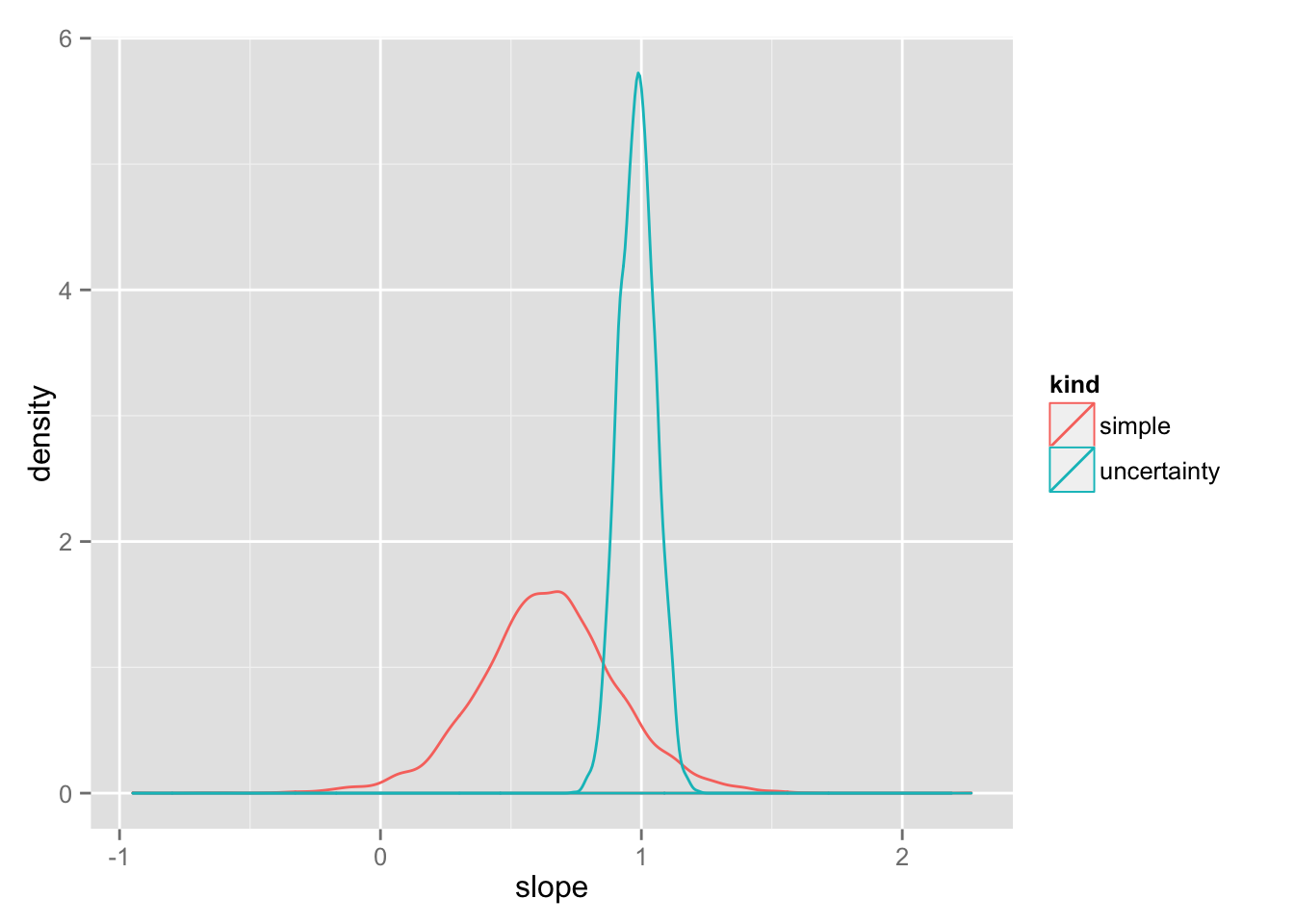``ggplot(df_coef, aes(intercept, color=kind)) + geom_density()``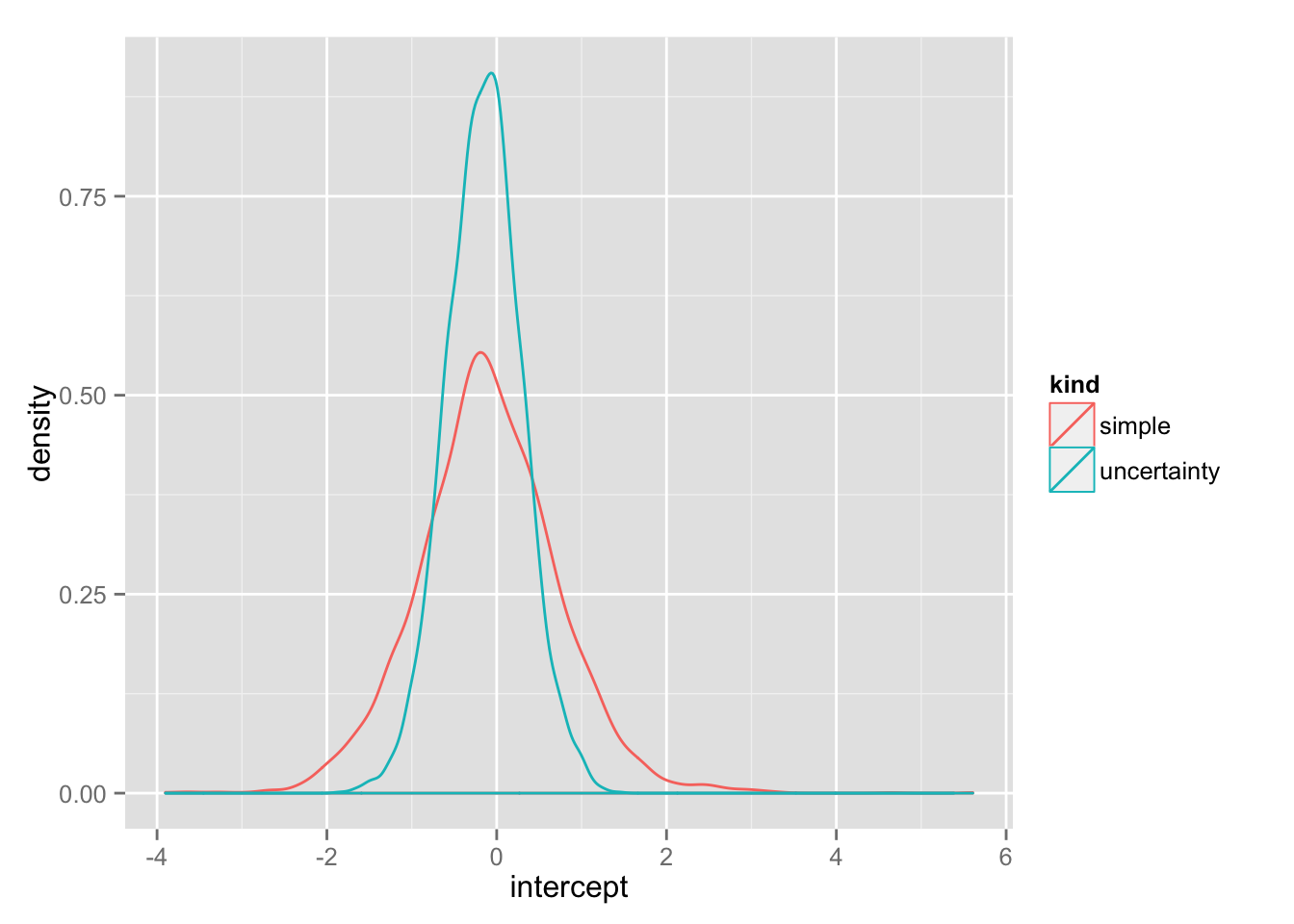Here are some sample fits:

``````samples <- sample.int(length(slopes), 50)
df_lines <- data.frame(slope=slopes[samples], intercept=intercepts[samples])

ggplot( data, aes(x, y) ) + geom_point() + geom_abline(data=df_lines, aes(slope=slope, intercept=intercept), color="grey") + coord_fixed() + geom_abline(color="red") + geom_smooth(method="lm", se=F)``````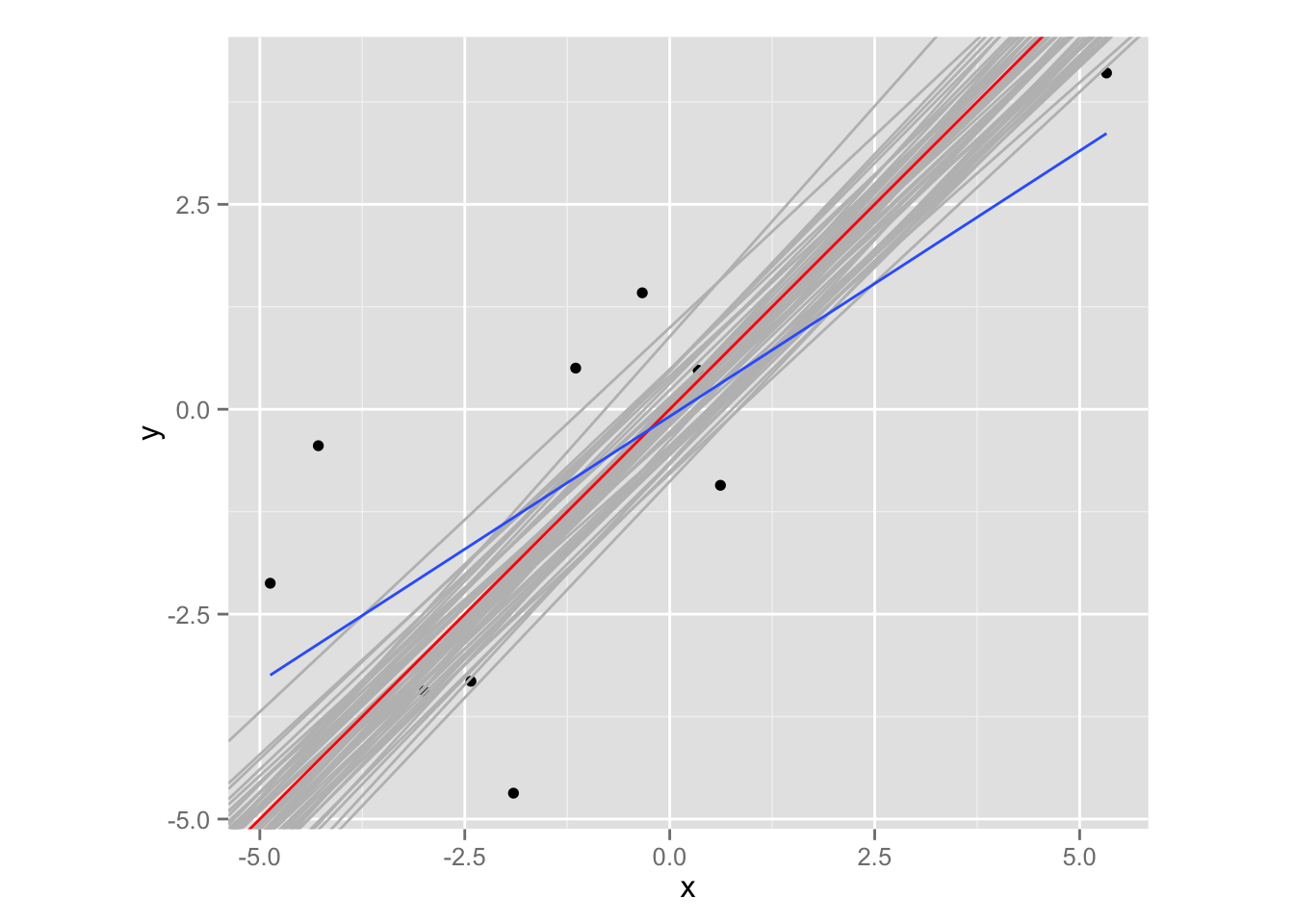And a view of the “moved” points:

``````xx <- do.call(cbind, extract(fit, "xx"))
yy <- do.call(cbind, extract(fit, "yy"))

df_points <- data.frame( x=c(x, xx[samples,]), y=c(y, yy[samples,]), kind = rep( c("measured", "inferred"), each=N), group = rep(1:N,2)  )

ggplot( df_points, aes(x, y, group=group)) + geom_point(aes(color=kind)) + geom_path() + geom_abline(color="green") + coord_fixed()``````